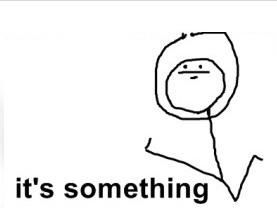# Doing some maths for last time of my life

Hi everyopne,

I have done my last math exercise for last time of my studies (I hope) :
first I passed MDI210 (with 10/20 but it’s something), meaning I don’t need maths UE anymore and…it’s something

Then I proposed maths formulas to resolve robots positions with only 3 beacons positions and relative distance between robots and beacons :

let’s assume three beacons, representing an orthogonal reference : P1 : origin, P2 : x axis, P3 : y axis
P4 robot position : what we are searching for. Lets assume Xn and Yn the coordinates of Pn.
Rn : the distance between Pn and P4 : Pn radio radius, position possibilities of the detected robot

X4 = ( R1^2 – R2^2 + X2^2 ) / 2 * X2
Y4 = ( R1^2 – R3^2 + X3^2 + Y3^2 ) / (2 * Y3 – (X3 / Y3) * X4 )

with robot on P4 with coordinates (X4, Y4)

What do you think about my incredible maths ? 😉

I also progressed on the IMU part, I understand the interest of using Stream in ChibiOS for debug messages because it is an abstract layer and I can used it on serial connection, or serial over usb of even with socket !
I also founded some MPU drivers on github that I will use / port on Chibios.

That’s all, good night 🙂### 5 comments to Doing some maths for last time of my life

•Alexis Polti

I’m a little dubious about the formula: if I understand correctly, you’re searching for the intersection of 3 circles. But what if (because of noise / errors on the measures) the circles don’t intersect on one point? By this I mean that each pair of circles will give you a point (2 in fact, but one of them can easily be eliminated if the beacons are placed at the edges of the playground). But the 3 points won’t probably be the same. So the 3 circles will rather give you a zone (a rounded triangle) in which the robot will be. So how will you treat this case?

Merry xMas and Happy New Year 🙂

•Alexis Polti

Error 404…

•perceval

Sorry we made some git branch -d 🙂
here is the new link but the algorithm may have changed by the time

•Alexis Polti